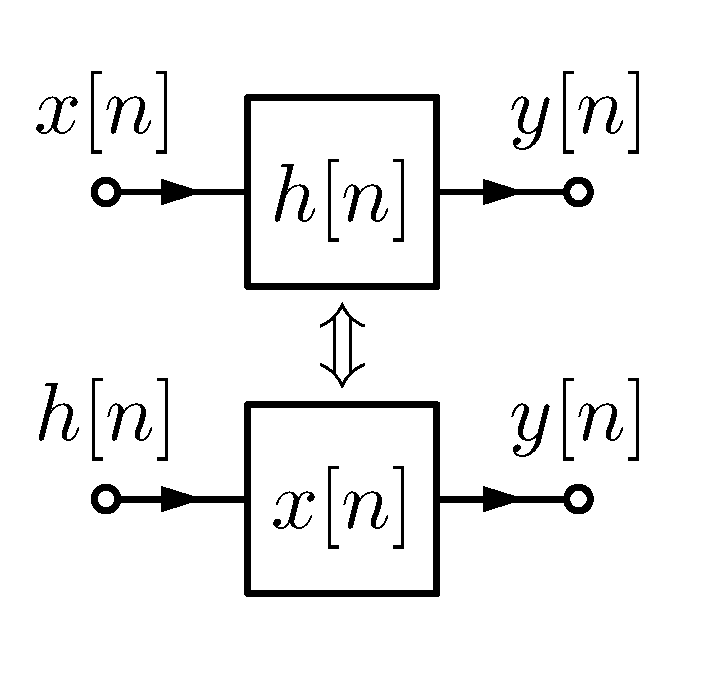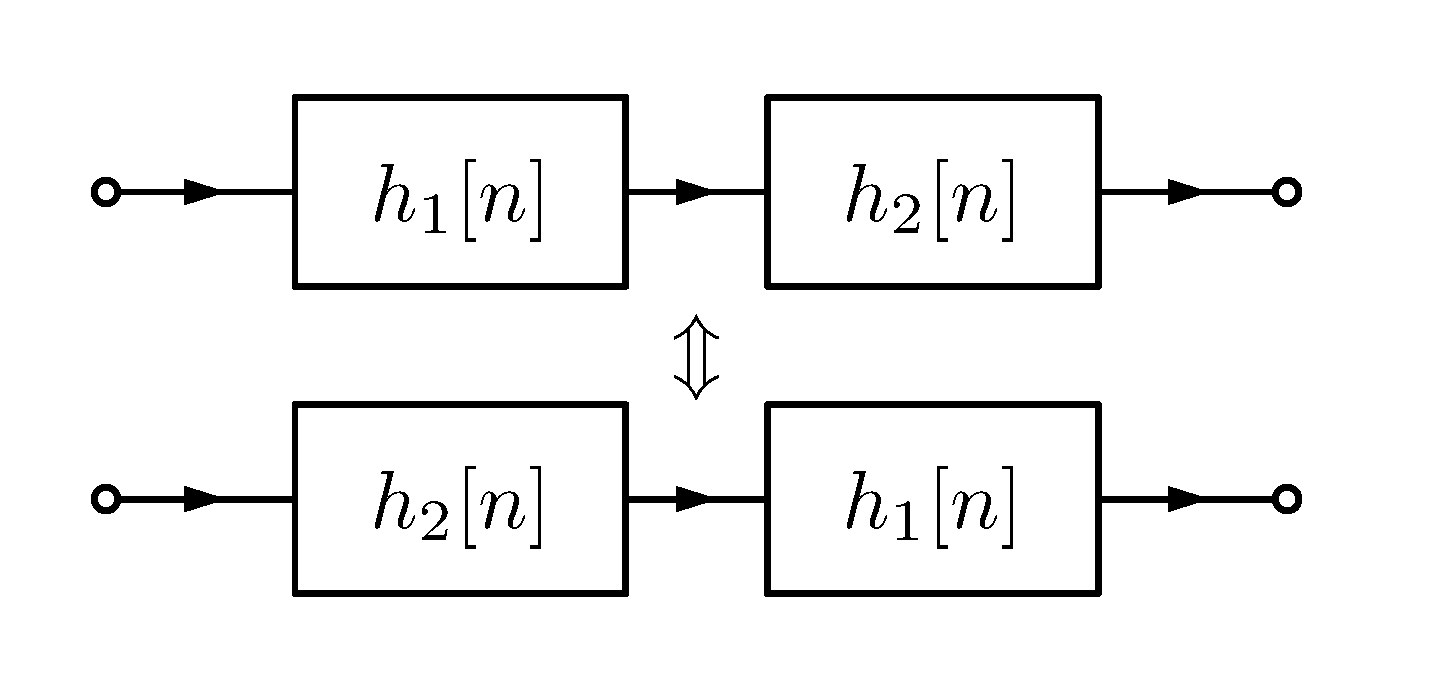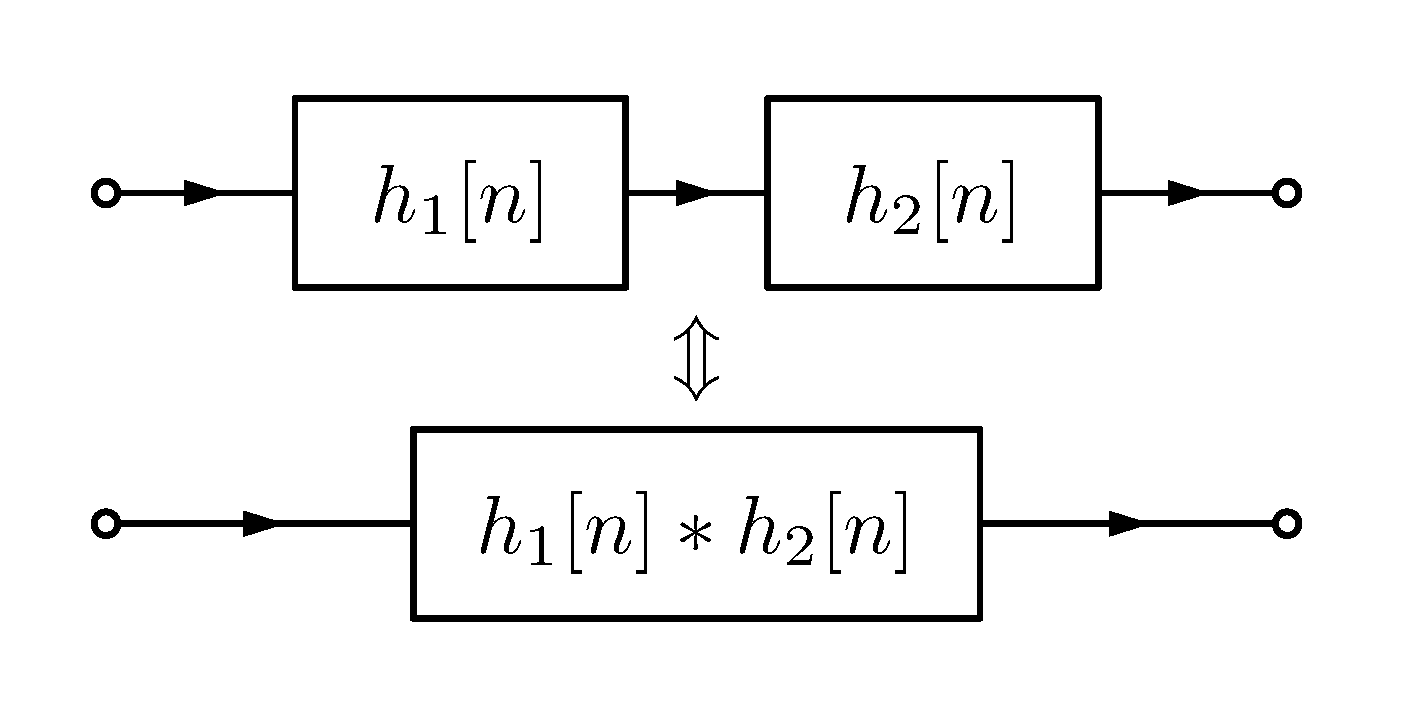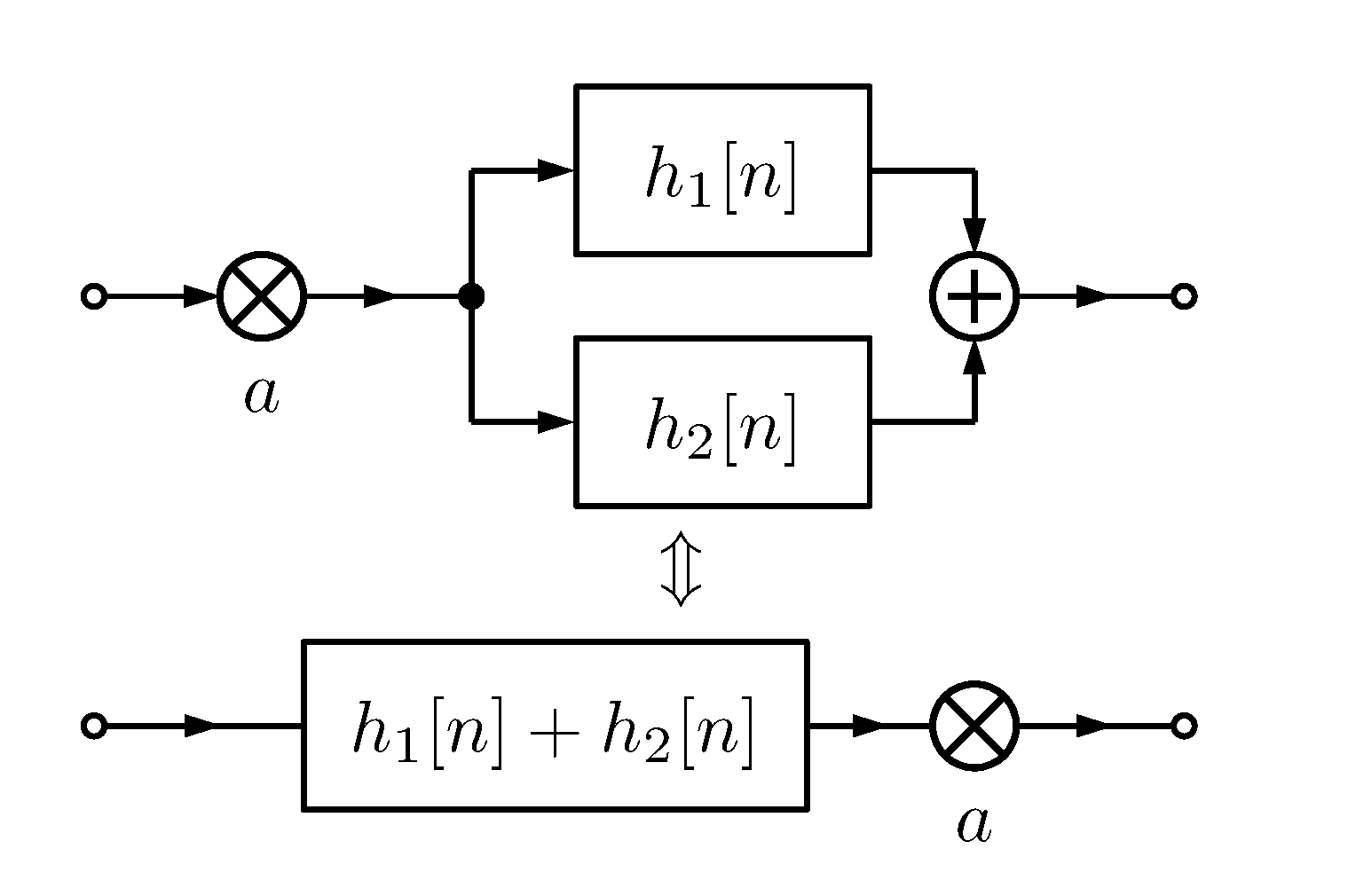Inspecting the mathematical properties of convolution leads to interesting conclusions regarding digital signal processing.

In the previous article we discussed the definition of the convolution operation. Now, it is time to look more closely at its mathematical properties in the context of digital signal processing.

### Recap

Let us recap the definition of the discrete convolution. With discrete signals $x[n], h[n]$ being square-summable, their convolution is defined as $x[n] \ast h[n] = \sum_{k=-\infty}^{\infty} x[k] h[n - k] = y[n], \quad n \in \mathbb{Z}. \quad (1)$ Convolution for continuous, square-integrable $x, h$ is defined as follows $x(t) \ast h(t) = \int \limits_{-\infty}^{\infty} x(\tau) h(t - \tau) d\tau, \quad t \in \mathbb{R}. \quad (2)$

In the following considerations we assume, that $x$ is some signal (e.g., an audio signal) and $h$, $h_1$, $h_2$ are impulse responses of some filters.

## Properties of convolution

• commutativity: $x \ast h = h \ast x$
• associativity: $x \ast (h_1 \ast h_2) = (x \ast h_1) \ast h_2$
• linearity:
$(\alpha x_1 + \beta x_2) \ast h = \alpha (x_1\ast h) + \beta (x_2 \ast h)$

(Here, $x$ and $h$ can be both discrete or both continuous.)

Their formulations and proofs are provided for the discrete as well as continuous cases.

### Commutativity

Commutativity of an operation means that its operands can be exchanged without affecting the result

$x \ast h = h \ast x. \quad (3)$

This property has a very interesting interpretation in the context of signal processing. It turns out, we can interpret a system’s impact on a signal as the signal’s impact on the system’s impulse response. In particular, the filtering operation can be viewed as if the input signal was filtering the filter’s impulse response (Figure 1). As we have already seen in the previous article, it is completely true: the output of a filter is a sum of its repeatedly scaled and delayed (=filtered) impulse response.Figure 1. Commutativity of convolution enables an exchange of filter with its input.

Another, even more profound interpretation of the commutativity of convolution is shown in Figure 2.Figure 2. Commutativity of convolution makes the ordering of filters in a series irrelevant.

The commutativity property means that we can exchange the order in which we apply filters (here represented by impulse responses $h_1[n]$ and $h_2[n]$). It doesn’t matter whether we filter input with $h_1$ and then with $h_2$ or the other way around; the result will be the same.

#### Proof for the discrete case

$x[n] \ast h[n] = \sum_{k=-\infty}^{\infty} x[k] h[n - k] = | k' = n-k; k = n - k'| \\ =\sum_{k'=-\infty}^{\infty} x[n-k'] h[k'] = \sum_{k'=-\infty}^{\infty} h[k'] x[n-k'] = h[n] \ast x[n]. \quad \Box$

#### Proof for the continuous case

$x(t) \ast h(t) = \int \limits_{-\infty}^{\infty} x(\tau) h(t - \tau) d\tau \\ = | \tau' = t - \tau; \tau = t - \tau'; d\tau' = -d\tau | \\ = - \int \limits_{\infty}^{-\infty} x(t - \tau') h(\tau') d\tau' = \int \limits_{-\infty}^{\infty} h(\tau') x(t - \tau') d\tau' \\= h(t) \ast x(t). \quad \Box$

Note the inversion of boundaries and the resulting change of the sign.

### Associativity

Associativity of an operation ensures that we can calculate the results of this operation in any order when given a couple of them in series $x \ast (h_1 \ast h_2) = (x \ast h_1) \ast h_2. \quad (4)$

A practical interpretation of this would be that a series of filters applied one after another constitutes an equivalent system to a system whose impulse response is a convolution of the filters’ impulse responses. So the impulse response of filters arranged in a series is a convolution of their impulse responses (Figure 3).Figure 3. Associativity of the convolution enables us to exchange successive filters with a single filter whose impulse response is a convolution of the initial filters’ impulse responses.

#### Proof for the discrete case

$x[n] \ast (h_1[n] \ast h_2[n]) = x[n] \ast \sum_{k=-\infty}^{\infty} h_2[k]h_1[n-k] \\= \sum_{l=-\infty}^{\infty}\sum_{k=-\infty}^{\infty} x[l]h_2[k]h_1[n-l-k] \\= \sum_{k=-\infty}^{\infty} h_2[k] \sum_{l=-\infty}^{\infty} x[l]h_1[n-k-l] \\= \sum_{k=-\infty}^{\infty} h_2[k] (x \ast h_1)[n-k] \\= ((x \ast h_1) \ast h_2) [n] \\= (x[n] \ast h_1[n]) \ast h_2[n]. \quad \Box$

#### Proof for the continuous case

$x(t) \ast (h_1(t) \ast h_2(t)) \\= x(t) \ast \int \limits_{-\infty}^{\infty} h_2(\tau) h_1(t - \tau) d\tau \\= \int \limits_{-\infty}^{\infty} x(\psi) \int \limits_{-\infty}^{\infty} h_2(\tau) h_1(t - \psi - \tau) d\tau d\psi \\= \int \limits_{-\infty}^{\infty} h_2(\tau) \int \limits_{-\infty}^{\infty} x(\psi)h_1(t - \tau - \psi) d \psi d \tau \\= \int \limits_{-\infty}^{\infty} h_2(\tau) (x \ast h_1)(t - \tau) d\tau = ((x \ast h_1) \ast h_2)(t) \\= (x(t) \ast h_1(t)) \ast h_2(t). \quad \Box$

### Linearity

The last property to be examined and proved is the linearity property of the convolution $a(x \ast (h_1 + h_2)) = (ax \ast h_1) + (ax \ast h_2) \quad (5)$ which consists of distributivity $x \ast (h_1 + h_2) = x \ast h_1 + x \ast h_2 \quad (6)$ and associativity with scalar multiplication $a (x \ast h) = (ax) \ast h. \quad (7)$

Distributivity means that filtering a signal with a superposition (a sum) of filters is equivalent to summing the results of filtering with the individual filters. It is as if the signal was filtered by them independently (in parallel). Thus, summing in digital signal processing corresponds to joining two independent paths of processing.

Thanks to this property we can filter the signal only once (with filters’ superposition) rather than run the signal through each filter separately and only then sum the outputs. As you can imagine, it can be a significant optimization advantage.

Sometimes, however, it may be easier to analyze a complex filter by splitting its impulse response into constituent impulse responses, effectively replacing a filter with a set of parallel filters. This is the idea underlying the filterbanks. It is also used in synthesis of a room impulse response for artificial reverberation (so-called modal decomposition).

Associativity with scalar multiplication simply means that it doesn’t matter if we scale the input signal (before filtering) or the output signal (after filtering); the resulting signal will be the same.

These equivalencies are depicted in Figure 4.Figure 4. Distributivity means that a signal filtered in parallel processing paths is effectively filtered by a superposition of these paths. Associativity with scalar multiplication enables us to scale either the input or the output of a system with an equal effect. These two properties determine the linearity of the convolution.

#### Proof for the discrete case

$a(x[n] \ast (h_1[n] + h_2[n])) \\= a \sum_{k=-\infty}^{\infty} x[k] (h_1[n-k] + h_2[n-k]) \\= \sum_{k=-\infty}^{\infty} ax[k]h_1[n-k] + ax[k]h_2[n-k] \\= \sum_{k=-\infty}^{\infty} ax[k]h_1[n-k] + \sum_{k=-\infty}^{\infty} ax[k]h_2[n-k] \\= (ax[n]) \ast h_1[n] + (ax[n]) \ast h_2[n]. \quad \Box$

#### Proof for the continuous case

$a(x(t) \ast (h1(t) + h2(t))) = a \int \limits_{-\infty}^{\infty} x(\tau) (h_1(t - \tau) + h_2(t - \tau)) d\tau \\= \int \limits_{-\infty}^{\infty} (ax(\tau) h_1(t - \tau) + ax(\tau)h_2(t - \tau))d \tau \\= \int \limits_{-\infty}^{\infty} a x(\tau) h_1(t - \tau) d\tau + \int \limits_{-\infty}^{\infty} ax(\tau) h_2(t - \tau) d\tau \\= (ax(t)) \ast h_1(t) + (ax(t)) \ast h_2(t). \quad \Box$

## Summary

In this article we reviewed the most important mathematical properties of the convolution, namely

• commutativity,
• associativity, and
• linearity.

These properties will prove themselves useful in our future considerations of convolution.

## Bibliography

 Convolution on Wikipedia. Retrieved: 09.03.2021.

 Alan V Oppenheim, Ronald W. Schafer Discrete-Time Signal Processing, 3rd Edition, Pearson 2010.

 Alan V. Oppenheim, Alan S. Willsky, with S. Hamid Signals and Systems, 2nd Edition, Pearson 1997.